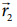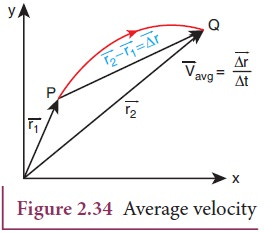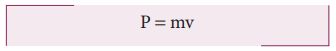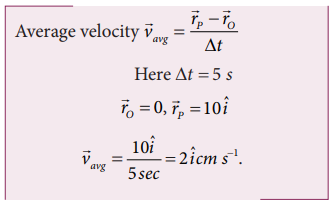Home | | Physics 11th std | Integral Calculus: Average velocity, Average speed, velocity, Momentum

# Integral Calculus: Average velocity, Average speed, velocity, Momentum

Integral Calculus: Average velocity, Average speed, velocity, Momentum

## Average velocity

Consider a particle located initially at point P having position vector. In a time interval the particle is moved to the point Q having position vector. The displacement vector is ∆=. This is shown in Figure 2.34.

The average velocity is defined as ratio of the displacement vector to the corresponding time intervalIt is a vector quantity. The direction of average velocity is in the direction of the displacement vector (Δ).

This is also shown in Figure 2.34.## Average speed

The average speed is defined as the ratio of total path length travelled by the particle in a time interval.## Instantaneous velocity or velocity

The instantaneous velocity at an instant t or simply ‘velocity’ at an instant t is defined as limiting value of the average velocity as Δ 0, evaluated at time t.

In other words, velocity is equal to rate of change of position vector with respect to time. Velocity is a vector quantity.In component form, this velocity isThe magnitude of velocity v is called speed and is given bySpeed is always a positive scalar. The unit of speed is also m

## Momentum

The linear momentum or simply momentum of a particle is defined as product of mass with velocity. It is denoted as. Momentum is also a vector quantity.The direction of momentum is also in the direction of velocity, and the magnitude of momentum is equal to product of mass and speed of the particle.In component form the momentum can be written asHere px = x component of momentum and is equal to mvx

py = y component of momentum and is equal to mvy

pz = z component of momentum and is equal to mvz

The momentum of the particle plays a very important role in Newton’s laws. The physical significance of momentum can be well understood by the following example.

Consider a butterfly and a stone, both moving towards you with the same velocity 5 m s-1. If both hit your body, the effects will not be the same. The effects not only depend upon the velocity, but also on the mass. The stone has greater mass compared to the butterfly. The momentum of the stone is thus greater than the momentum of the butterfly. It is the momentum which plays a major role in explaining the ‘state’ of motion of the object.

The unit of the momentum is kg m s-1

### Solved Example Problems for Average speed

Example 2.20

Consider an object travelling in a semi-circular path from point O to point P in 5 second, as is shown in the Figure. Calculate the average velocity and average speed.SolutionThe average velocity is in the positive x direction.

The average speed = total path length / time taken (the path is semi-circular)Note that the average speed is greater than the magnitude of the average velocity.

### Solved Example Problems Instantaneous velocity or velocity

Example 2.21

The position vector of a particle is givenCalculate the velocity and speed of the particle at any instant t

Calculate the velocity and speed of the particle at time t = 2 s

SolutionNote that the particle has velocity components along x and y direction. Along the z direction the position has constant value (-5) which is independent of time. Hence there is no z-component for the velocity.

Example 2.22

The velocity of three particles A, B, C are given below. Which particle travels at the greatest speed?Solution

We know that speed is the magnitude of the velocity vector. Hence,The particle C has the greatest speed.

Example 2.23

Two cars are travelling with respective velocities=10ms-1 along east and=10ms-1  along west What  are the speeds of the cars?

Solution

Both cars have the same magnitude of velocity. This implies that both cars travel at the same speed even though they have velocities in different directions. Speed will not give the direction of motion.### Solved Example Problems Momentum

Example 2.24

Consider two masses of 10 g and 1 kg moving with the same speed 10 m s-1. Calculate the magnitude of the momentum.

Solution

We use p = mv

For the mass of 10 g, m = 0.01 kgThus even though both the masses have the same speed, the momentum of the heavier mass is 100 times greater than that of the lighter mass.

Tags : with Solved Example Problems , 11th Physics : UNIT 2 : Kinematics
Study Material, Lecturing Notes, Assignment, Reference, Wiki description explanation, brief detail
11th Physics : UNIT 2 : Kinematics : Integral Calculus: Average velocity, Average speed, velocity, Momentum | with Solved Example Problems